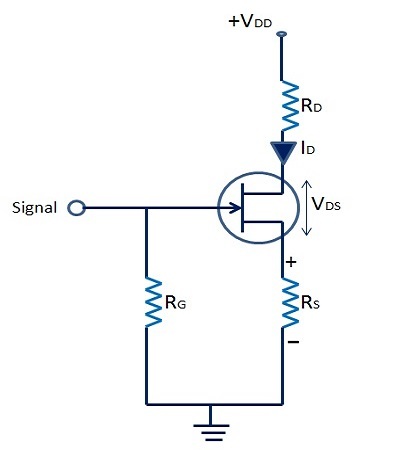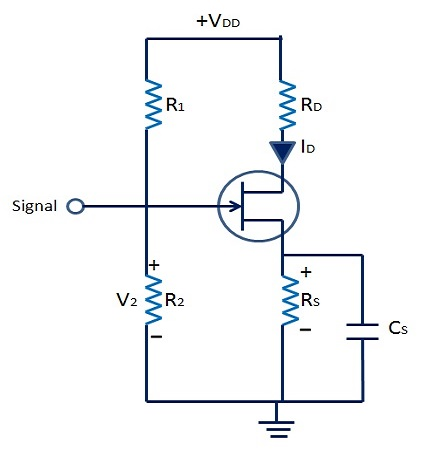# Semiconductor Devices - JFET Biasing

There are two methods in use for biasing the JFET: Self-Bias Method and Potential Divider Method. In this chapter, we will discuss these two methods in detail.

## Self-Bias Method

The following figure shows the self-bias method of n-channel JFET. The drain current flows through Rs and produces the required bias voltage. Therefore, Rs is the bias resistor.Therefore, voltage across bias resistor,

$$V_s = I_{DRS}$$

As we know, gate current is negligibly small, the gate terminal is at DC ground, VG = 0,

$$V_{GS} = V_G - V_s = 0 - I_{DRS}$$

Or $V_{GS} = -I_{DRS}$

VGS keeps gate negative w.r.t. to the source.

## Voltage Divider Method

The following figure shows voltage divider method of biasing the JFETs. Here, resistor R1 and R2 form a voltage divider circuit across drain supply voltage (VDD), and it is more or less identical to the one used in transistor biasing.The voltage across R2 provides necessary bias −

$$V_2 = V_G = \frac{V_{DD}}{R_1 + R_2} \times R_2$$

$= V_2 + V_{GS} + I_D + R_S$

Or $V_{GS} = V_2 - I_{DRS}$

The circuit is so designed that VGS is always negative. The operating point can be found using the following formula −

$$I_D = \frac{V_2 - V_{GS}}{R_S}$$

and $V_{DS} = V_{DD} - I_D(R_D + R_S)$